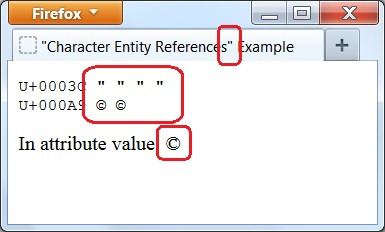Using Numeric Character References

This section describes how numeric character references can be used in HTML documents. Numeric character references allow you to represent any Unicode code points in HTML documents.

HTML5 also supports numeric character references to represent any Unicode code points. You need to remember the following notes when using them:

1. A numeric character reference can be written in decimal format as "&#nnnn;", where nnnn is the code point in decimal digits. For example, "&60;" is a numeric character reference to Unicode code point of U+0003C for character "&lt;".

2. A numeric character reference can be written in hexadecimal format as "&#xhhhh;", where hhhh is the code point in hexadecimal digits. For example, "&x3c;" is a numeric character reference to Unicode code point of U+0003C for character "&lt;".

3. Numeric character references can be used in element contents of all HTML elements except "script" and "style" elements.

6. Numeric character references in "svg" and "math" element contents must follow XML rules.

7. Numeric character references can also be used in attribute values.

Here is example HTML document that shows you how to use character entity references:

```<!DOCTYPE html>
<!-- HTM_Numeric_Character_References_Example.html
-->
<html>
<!-- Numeric Character References in "title" element -->
<title>&#34;Character Entity References&#x22; Example</title>
<body>

<!-- Numeric Character References in element content -->
<pre>
U+0003C &#34; &#x22; &#034; &#x0022;
U+000A9 &#169; &#xA9;
</pre>

<!-- Numeric Character References in attribute value -->
<img src="image.gif" alt="In attribute value: &#xA9;">